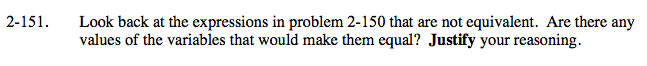### Home > AC > Chapter 13 > Lesson 13.RE3-S > Problem2-151

2-151.

Look back at the expressions in problem 2-150 that are not equivalent. Are there any values of the variables that would make them equal? Justify your reasoning. Homework Help ✎For parts (d) and (f) try setting the expressions or equations equal to each other, then simplify, and explain the meaning of the results.

In part (d), the values a = 0 or b = 0 would make the two expressions equal.

In part (e), x ≠ 0 because it is not in the denominator.

x = 1 will make the expressions equal.